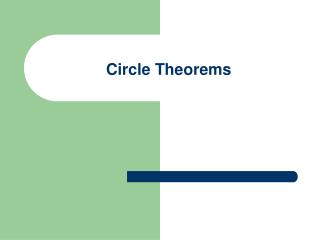DownloadDownload PresentationCircle Theorems

# Circle Theorems

Download Presentation## Circle Theorems

- - - - - - - - - - - - - - - - - - - - - - - - - - - E N D - - - - - - - - - - - - - - - - - - - - - - - - - - -
##### Presentation Transcript

1. Circle Theorems

2. Properties • Circles • Circle Theorems • Angles Subtended on the Same Arc • Angle in a Semi-Circle with Proof • Tangents • Angle at the Centre with Proof • Alternate Segment Theorem with Proof • Cyclic Quadrilaterals

3. Circles • A circle is a set of points which are all a certain distance from a fixed point known as the centre (O). • A line joining the centre of a circle to any of the points on the circle is known as a radius (AO & BO).

4. Circles • The circumference of a circle is the length of the circle. The circumference of a circle = 2 × π × the radius. • The red line in thisdiagram is called a chord. It divides the circle into a major segment and a minor segment.

5. Angles Subtended on the Same Arc • Angles formed from two points on the circumference are equal to other angles, in the same arc, formed from those two points.

6. Angle in a Semi-Circle • Angles formed by drawing lines from the ends of the diameter of a circle to its circumference form a right angle. So C is a right angle.

7. Proof • We can split the triangle in two by drawing a line from the centre of the circle to the point on the circumference our triangle touches. • We know that each of the lines which is a radius of the circle (the green lines) are the same length. Therefore each of the two triangles is isosceles and has a pair of equal angles.

8. Proof • But all of these angles together must add up to 180°, since they are the angles of the original big triangle. • Therefore x + y + x + y = 180°, in other words 2(x + y) = 180°. And so x + y = 90°.

9. Tangents • A tangent to a circle is a straight line which touches the circle at only one point • A tangent to a circle forms a right angle with the circle's radius, at the point of contact of the tangent.

10. Tangents • If two tangents are drawn on a circle and they cross, the lengths of the two tangents (from the point where they touch the circle to the point where they cross) will be the same.

11. Angle at the Centre • The angle formed at the centre of the circle by lines originating from two points on the circle's circumference is double the angle formed on the circumference of the circle by lines originating from the same points. i.e. a = 2b.

12. Proof • OA = OX since both of these are equal to the radius of the circle. • The triangle AOX is therefore isosceles and so ∠OXA = a. Similarly, ∠OXB = b.

13. Proof • Since the angles in a triangle add up to 180°, we know that ∠XOA = 180° - 2a • Similarly, ∠BOX = 180° - 2b • Since the angles around a point add up to 360°, we have that ∠AOB = 360° - ∠XOA - ∠BOX • = 360° - (180° - 2a) - (180° - 2b) • = 2a + 2b = 2(a + b) = 2 ∠AXB

14. Alternate Segment Theorem • The alternate segment theorem shows that the red angles are equal to each other and the green angles are equal to each other.

15. Proof • A tangent makes an angle of 90° with the radius of a circle, so we know that ∠OAC + x = 90°. • The angle in a semi-circle is 90°, so ∠BCA = 90°.

16. Proof • The angles in a triangle add up to 180°, so ∠BCA + ∠OAC + y = 180°. • Therefore 90° + ∠OAC + y = 180° and so ∠OAC + y = 90°. • But OAC + x = 90°, so ∠OAC + x = ∠OAC + y. • Hence x = y.

17. Cyclic Quadrilaterals • A cyclic quadrilateral is a four-sided figure in a circle, with each vertex (corner) of the quadrilateral touching the circumference of the circle. The opposite angles of such a quadrilateral add up to 180°.